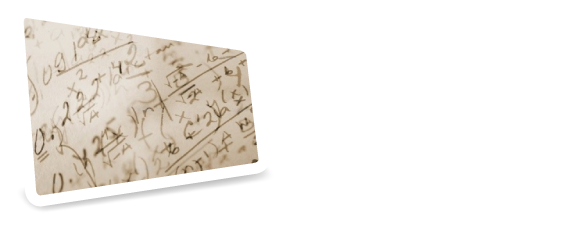# List of acoustics modules

dBWaves provides a large variety of acoustics calculation modules. With them you can develop acoustic designs online without the need to install any software on your computer. You just need a web browser. Read more about the benefits here.

#### Outdoors propagation

 Noise map Allows to calculate sound propagation outdoors using an extensive library of sources and objects like buildings and barriers. With an intuitive and easy to use interface. Integrated with Google Maps. Based on ISO-9613 "Attenuation of sound during propagation outdoors".

#### Insulation calculations

 R, Single Wall Calculates the sound reduction index R and the weighted sound reduction index Rw (ISO 717-1) for a single wall, using materials from the dBWaves  database or from the user database. R, Double Wall Calculates the sound reduction index R and the weighted sound reduction index Rw (ISO 717-1) for a double wall, using materials from the dBWaves database or from the user database. R, Composite Wall Calculate the sound reduction index R and the weighted sound reduction index Rw (ISO 717-1) for a wall made ​​of several different surfaces, using designs (dBWaves or user) or user projects. R + Flanking transmission Allows to calculate upper and lower limit values of the sound reduction index R and the weighted sound reduction index Rw (ISO 717-1) between two rooms, considering the effect of indirect transmission (based on EN 12354, ISO 15712) using designs (dBWaves or user) or user projects. Ln, R, Impact Noise Calculates the Normalized impact SPL Ln  and the corresponding Weighted normalized SPL Lnw (ISO 717-2), using materials from the dBWaves database or from the user database. Ln + Flanking Transmission Allows to calculate upper and lower limit values of the Normalized impact SPL Ln, the corresponding Weighted normalized SPL Lnw (ISO 717-2), the sound reduction index R and the weighted sound reduction index Rw (ISO 717-1) between two rooms, considering the effect indirect transmission (based on EN 12354, ISO 15712) using designs (dBWaves or user) or user projects. Noise barrier Allows to calculate the attenuation achieved with a noise barrier in the frequency range between 50 and 5000 Hz. The calculation is based on ISO 9613, which considers also the effect of lateral diffraction and transmission through the barrier. Spectral attenuation Allows to apply the attenuation or sound reduction index of any existing project to a particular spectrum. The user can define the weighting curve used for data entry and presentation of results (Lineal, A or C).

#### Absorption calculations

 Absorption of porous layers Calculates the sound absorption coefficient α and the weighted sound absorption coefficient αw (ISO 11654) for porous layers of absorbent material (e.g. polyurethane foam, glass wool, mineral wool, etc.). Membrane resonator Calculates the sound absorption coefficient α and the weighted sound absorption coefficient αw (ISO 11654) of membrane or plate resonators. Perforated resonators Calculates the sound absorption coefficient α and the weighted sound absorption coefficient αw (ISO 11654) of resonators with a perforated plates. Slit resonators Calculate the sound absorption coefficient α and the weighted sound absorption coefficient αw (ISO 11654) of slit resonators. Composite absorption & Rev. time Calculate the sound absorption coefficient α and the weighted sound absorption coefficient αw (ISO 11654) of walls composed by several different aborbing surfaces using designs (dBWaves or user) or user projects. Furthermore, by assigning a volume, estimates the reverberation time.

#### Mufflers

 Splitter silencer Allows to calculate the attenuation of a splitter type muffler in third octave bands between 50 and 5000 Hz. It also allows to estimate the pressure drop for a given discharge value, according to the ISO 14163.